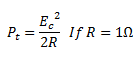# Explain the Difference between Narrow band FM and Wide band FM

## Types of FM

The FM systems are basically classified into following two types :

1. Narrow band FM
2. Wide band FM / Broadband FM

## Narrow Band FM

A narrow band FM is the FM wave with a small bandwidth .

The modulation index mof narrow band FM is small as compared to one radian . Hence, the spectrum of narrow band FM consists of the carrier and upper sideband and a lower sideband .

For small values of m, the values of the j coefficients are as under :

J0(mf) = 1,

J1(mf) = mf/2

Jn(mf) = 0 for n > 1

Hence, a narrow band FM wave can be expressed mathematically as under,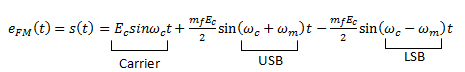The (-) sign associated with the LSB represents a phase shift of  180o.

Practically, the narrow band FM systems have  mless than 1 . The maximum permissible frequency deviation is restricted to about 5 kHz .

This system is used in FM mobile communications such as police wireless, ambulances, taxicabs etc .

### Analysis of Narrow band FM

As we know, the expression for instantaneous frequency of FM wave is given as :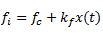Where, x(t) is the modulating signal .

The term kx(t) represents the frequency deviation . The constant kf  will control the deviation . For small values of  k, the frequency deviation is small and the spectrum of FM signal has a narrow band . Hence, it is called as the narrow band FM .

Let us consider the expression for FM wave as under :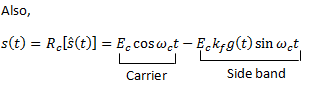This is the expression for narrow band FM .

Fig.1 shows the generation of narrow band FM using balanced modulator .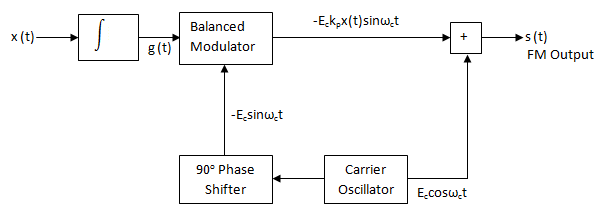Fig. 1

## Wideband FM

For large values of modulation index m, the FM wave ideally contains the carrier and an infinite number of sidebands located symmetrically around the carrier.

Such a FM wave has infinite bandwidth and hence called as wideband FM.

The modulation index of wideband FM is higher than 1.

The maximum permissible deviation is 75 kHz and it is used in the entertainment broadcasting applications such as FM radio, TV etc.

### Frequency Spectrum of a Wideband FM wave

The expression for the wideband FM is complex since it is sine of sine function.

The only way to solve this equation is by using the Bessel functions. By using the Bessel functions the equation for wideband FM wave can be expanded as follows :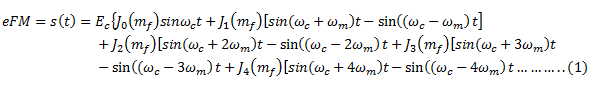Looking at equation (1), we can conclude the following points:

1. The FM wave consists of carrier. The first term in equation(1) represents the carrier.
2. The FM wave ideally consists of infinite number of sidebands. All the terms except the first one are sidebands.
3. The amplitudes of the carrier and sidebands is dependent on the J coefficients.
4. As the values of J coefficients are dependent on the modulation index  m, the modulation index determines how many sideband components have significant amplitudes as shown in fig.2 below.
5. Some of the J coefficients can be negative. Therefore, there is a 180phase shift for that particular pair of sidebands.
6. The carrier component does not remain constant. As J0(mf) is varying the amplitude of the carrier will also vary. However, the amplitude of FM wave will remain constant.
7. For certain values of modulation index, the carrier component will disappear completely. These values are called eigen values.
8. In FM, the total transmitted power always remains constant. It is not dependent on the modulation index. The reason for this is that the amplitude of the FM signal i.e.  Eis always constant. AND the power transmitted is given by,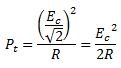Where E= peak amplitude of FM wave

Therefore,